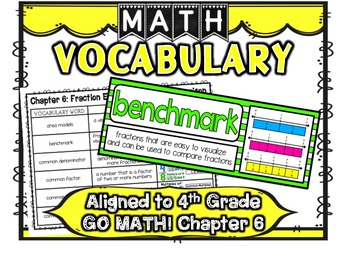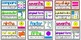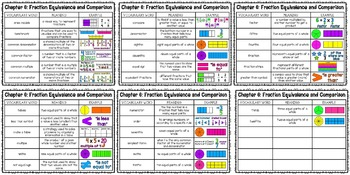Math Vocabulary Cards Aligned to 4th Grade GO Math! Chapter 6Subject
Resource Type
Product Rating
File Type

PDF (Acrobat) Document File

19 MB|46 pages
Share
Product Description
This product is aligned to GO Math! Grade 4 Chapter 6 Fraction Equivalence and Comparison. Vocabulary cards and student notebook pages include the vocabulary word, meaning of the word and a visual example of the meaning. Simply print and laminate the word wall cards and post on your math word wall! Simply print the student notebook pages and have students paste them in their math notebook or journal as a great reference tool and method for building their mathematical vocabulary!

Word List:
area models
benchmark fractions
common denominator
common factor
common multiple
common numerator
compare
denominator
eighths
elevenths
equal sign
equivalent fractions
factor
fifths
fourths
fraction
fraction strips
greater than symbol
halves
less than symbol
make a table
multiple
ninths
not equal sign
numerator
order
sevenths
simplest form
sixths
tenths
thirds
twelfths

Total Pages
46 pages